A very efficient method of forecasting one variable is to find a related variable that leads it by one or more time intervals. We use cross correlation approach here. Second approach, is to use information about the sales of similar products in the past. Where we study the bass curve. A third strategy is to make extrapolations based on present trends continuing.

We study first two approaches in this chaper.

## Australian Bureau of Statistics

Label: “Approvals” Period: Mar 1996 to Sep 2006 Data: values Any Transformation in the Data: Mean Type: Total Dwellings Approved Unit: Number Frequency: Quarterly Start of Frequency: Mar- May *End of Frequency: Jul-Sep

Label: “Activity” Period: Mar 1996 to Sep 2006 Data: values Any Transformation in the Data: Mean Type: Value of Work Done Unit: Miilions of Australian Dollars Frequency: Quarterly Start of Frequency: Mar- May *End of Frequency: Jul-Sep

Build.dat <- read.table("ts/ApprovActiv.dat", header=T) ; attach(Build.dat)
App.ts <- ts(Approvals, start = c(1996,1), freq=4)
Act.ts <- ts(Activity, start = c(1996,1), freq=4)
ts.plot(App.ts, Act.ts, lty = c(1,3))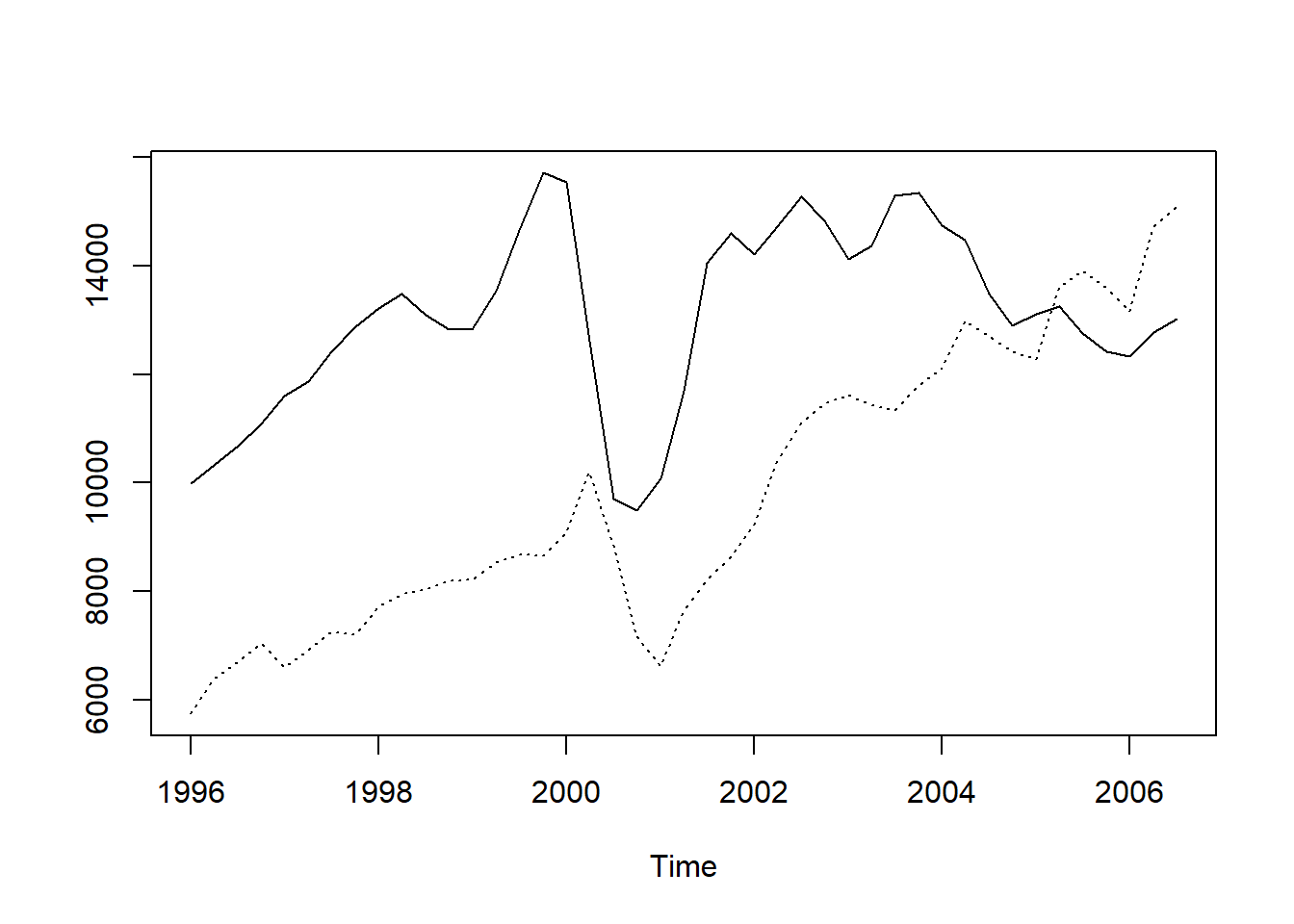We can see that Acvity lags one quarter behind approvals. Or Approvals lead the building activity by one quarter.

The Cross Correlation function can be used to quantify this relationship.

## ts.union : for Cross Correlation Correlogram

We draw it as follows:

acf(ts.union(App.ts,Act.ts))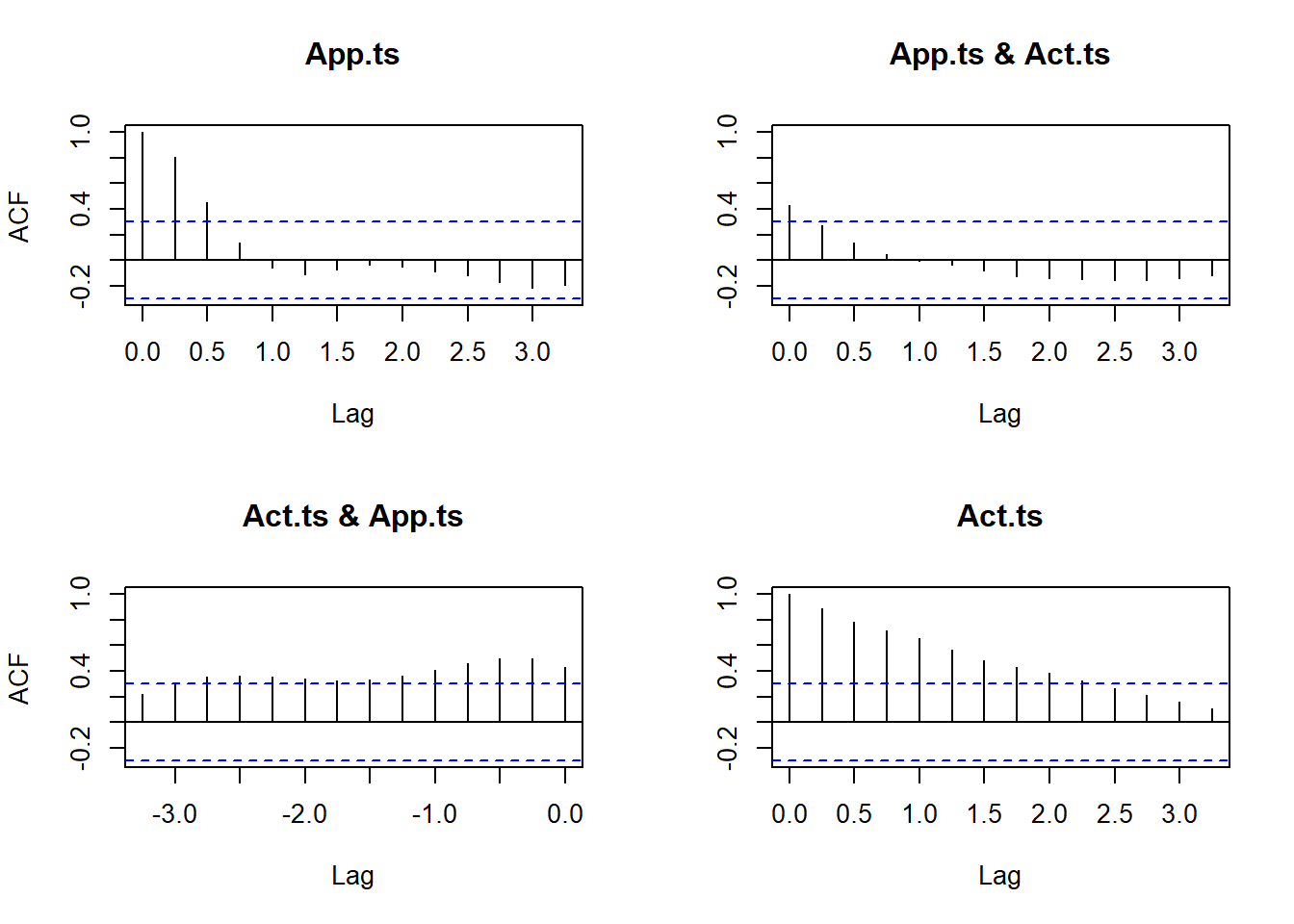Here we see in the upper right graph between App.ts and Act.ts that the cross correlation is about 0.35.Lets see the cross correlation after removing trend and seasonality. The left bottom graph is between Act.ts and App.ts

 app.ran <- decompose(App.ts)$random app.ran.ts <- window (app.ran, start = c(1996, 3) ) act.ran <- decompose (Act.ts)$random
act.ran.ts <- window (act.ran, start = c(1996, 3) )

Now we plot acf of cross correlation. Remember to take the action about NA Values, it passes through missing values.

acf(ts.union(app.ran.ts,act.ran.ts),na.action=na.pass)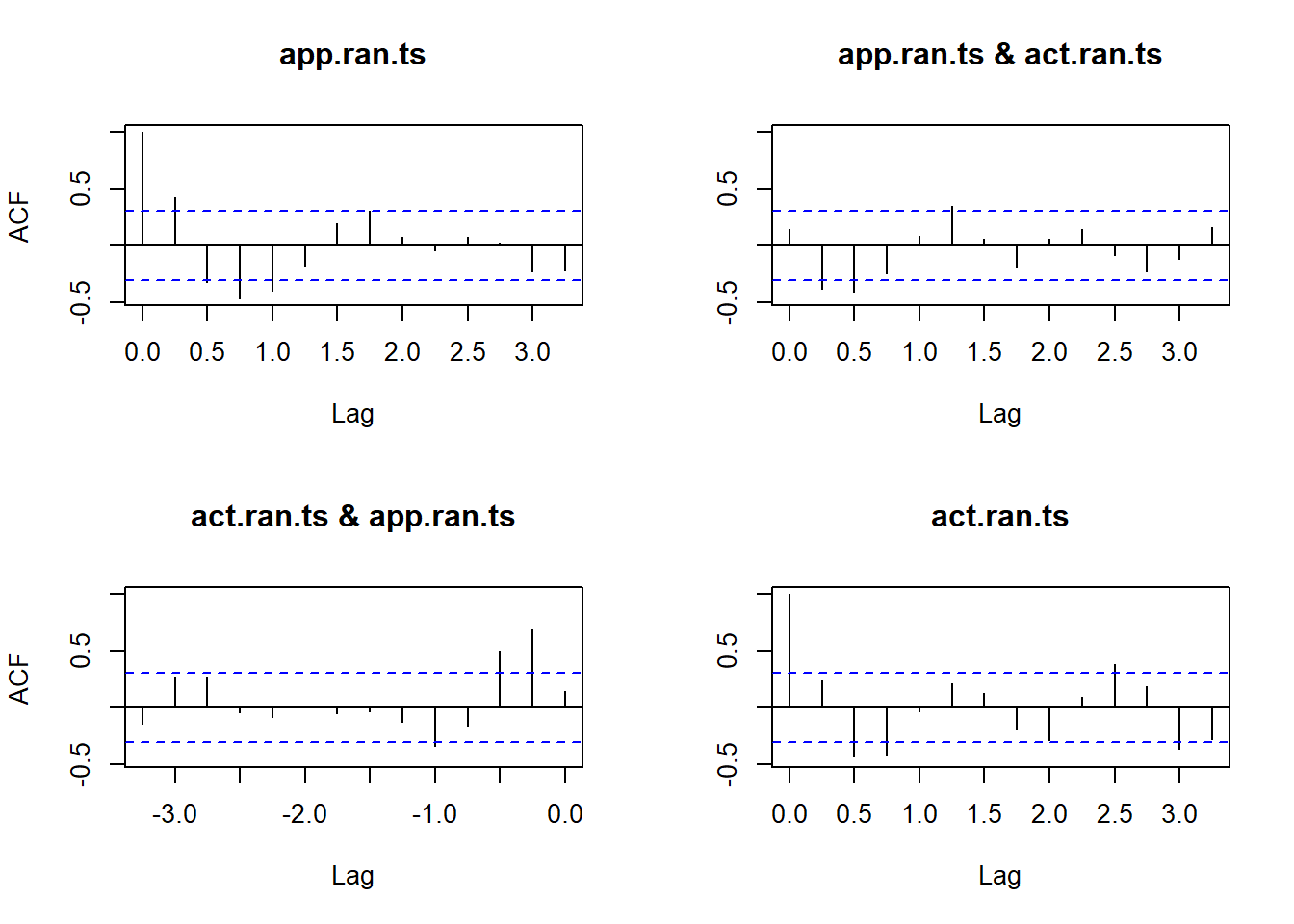## CCF plot

ccf(app.ran.ts,act.ran.ts,na.action=na.pass)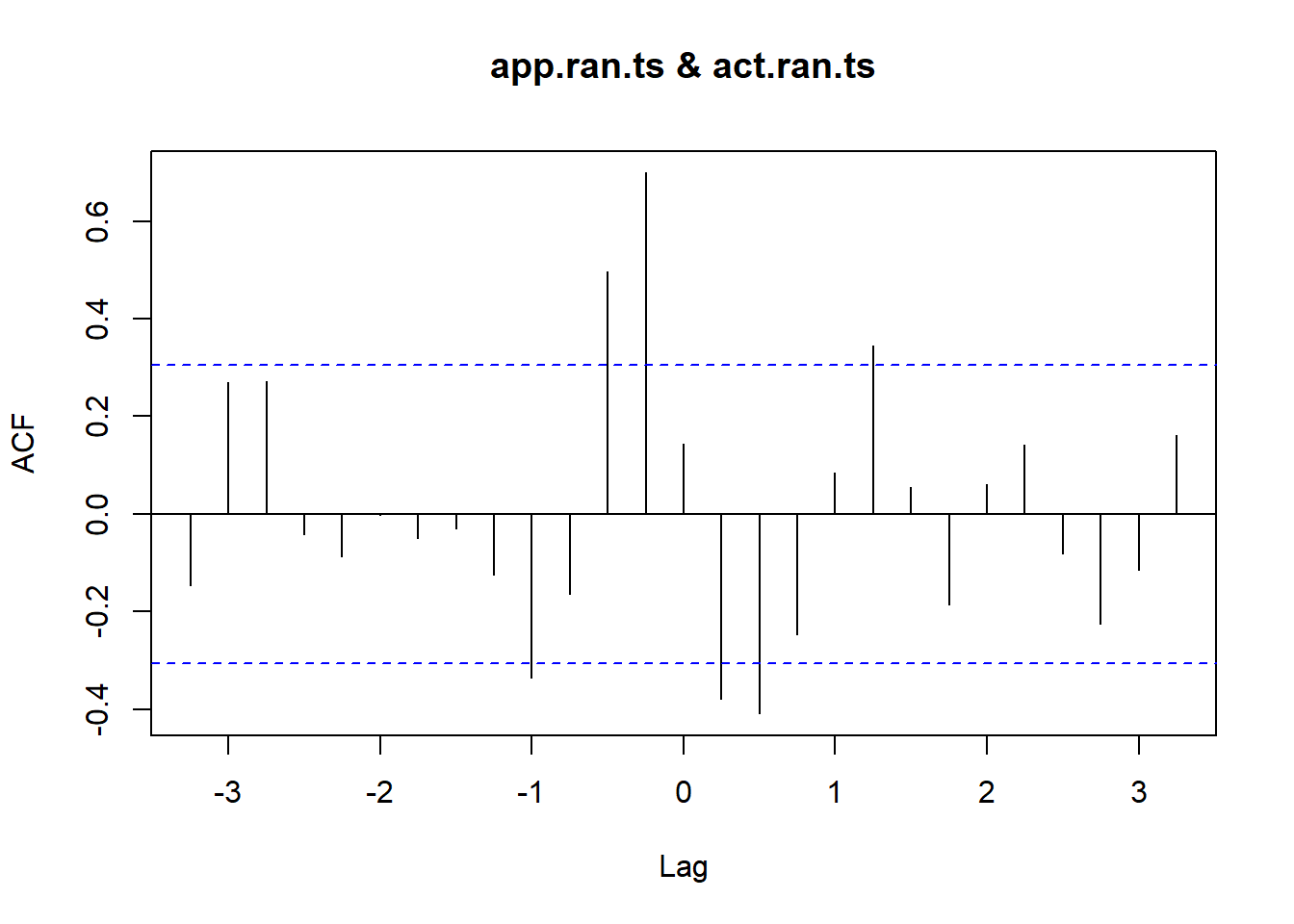You can print the values using print()function

print(ccf(app.ran.ts,act.ran.ts,na.action=na.pass))##
## Autocorrelations of series 'X', by lag
##
##  -3.25  -3.00  -2.75  -2.50  -2.25  -2.00  -1.75  -1.50  -1.25  -1.00
## -0.147  0.271  0.272 -0.042 -0.087 -0.002 -0.050 -0.031 -0.124 -0.336
##  -0.75  -0.50  -0.25   0.00   0.25   0.50   0.75   1.00   1.25   1.50
## -0.164  0.497  0.699  0.144 -0.380 -0.409 -0.247  0.084  0.346  0.056
##   1.75   2.00   2.25   2.50   2.75   3.00   3.25
## -0.187  0.060  0.142 -0.080 -0.225 -0.115  0.161

** Bass Model Please refere to the theory [here] (https://myanalyticsnotes.blogspot.com/2020/03/bass-model-for-forecasting.html) Let us say we are given the sales of VCR from 79 -89. We are to fit a bass curve between 80-89 ( we use 79 as t-1) (T79)

T79 <- 1:10

For plotting the curve we divide it every year in 10 parts by dividing by 10 ( Tdelt)

Tdelt <- (1:100)/10

Let sales be as follows:

Sales <- c(840,1470,2110,4000,7590,10950,10530,9470,7790,5890)

Now we calculate as follows: NLS- Non linear least square uses a relative-offset convergence criterion that compares the numerical imprecision at the current parameter estimates to the residual sum-of-squares ** NLS functions to obtain three parameters from one equation

Cusales <- cumsum(Sales)
Bass.nls <- nls(Sales ~ M * ( ((P+Q)^2 / P) * exp(-(P+Q) * T79) ) /(1+(Q/P)*exp(-(P+Q)*T79))^2, start = list(M=60630, P=0.03, Q=0.38))
summary(Bass.nls)
##
## Formula: Sales ~ M * (((P + Q)^2/P) * exp(-(P + Q) * T79))/(1 + (Q/P) *
##     exp(-(P + Q) * T79))^2
##
## Parameters:
##    Estimate Std. Error t value Pr(>|t|)
## M 6.798e+04  3.128e+03   21.74 1.10e-07 ***
## P 6.594e-03  1.430e-03    4.61  0.00245 **
## Q 6.381e-01  4.140e-02   15.41 1.17e-06 ***
## ---
## Signif. codes:  0 '***' 0.001 '**' 0.01 '*' 0.05 '.' 0.1 ' ' 1
##
## Residual standard error: 727.2 on 7 degrees of freedom
##
## Number of iterations to convergence: 8
## Achieved convergence tolerance: 7.323e-06

Thus we have got the value of M, P and Q as M= 679800, p=0.006 and q = 0.64 respectively. Now we can plot the curve

Bcoef <- coef(Bass.nls)
m <- Bcoef
p <- Bcoef
q <- Bcoef
ngete <- exp(-(p+q) * Tdelt)
Bpdf <- m * ( (p+q)^2 / p ) * ngete / (1 + (q/p) * ngete)^2
plot(Tdelt, Bpdf, xlab = "Year from 1979",
ylab = "Sales per year", type='l')
points(T79, Sales)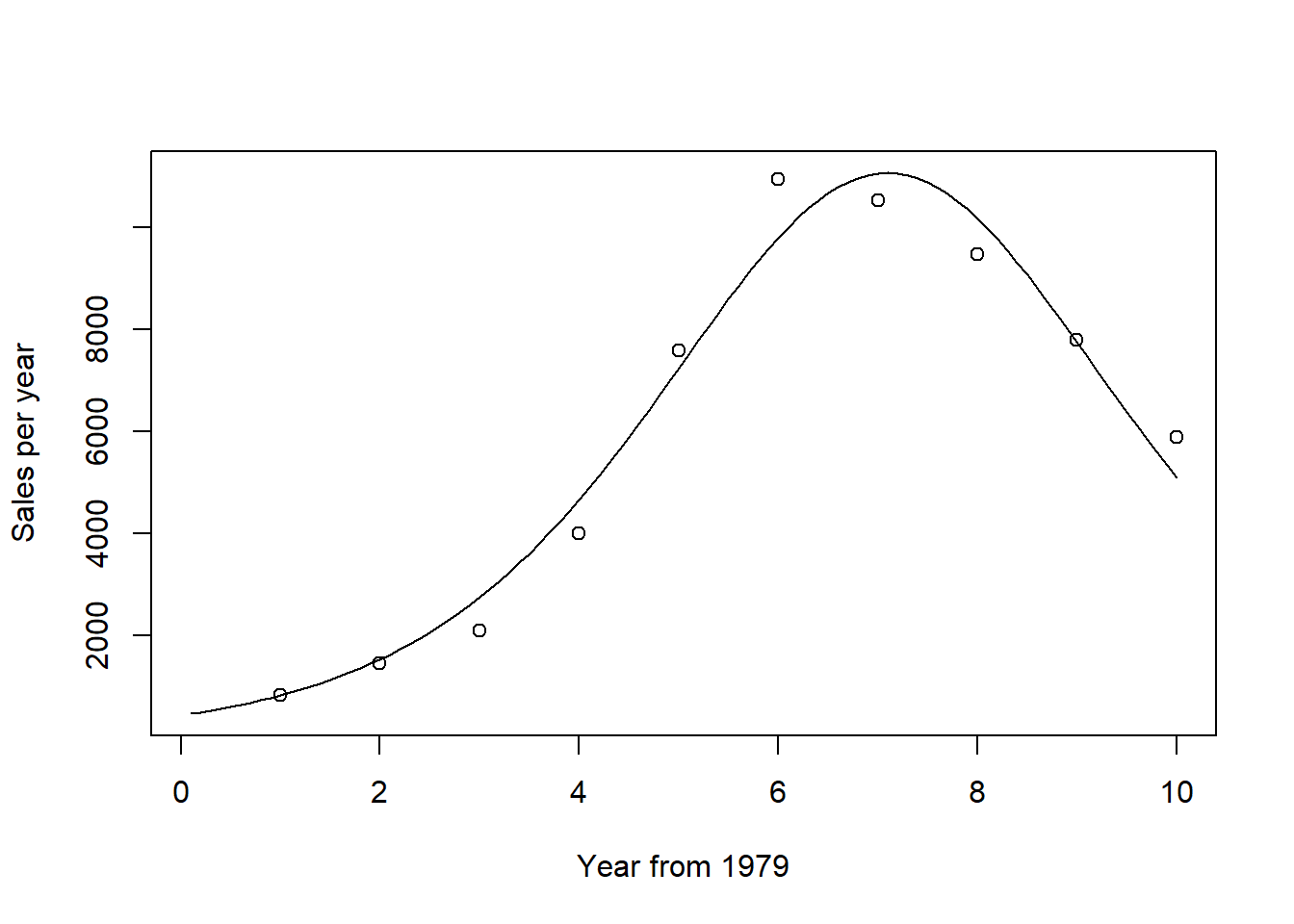We can plot cumulative Sales as follows:

Bcdf <- m * (1 - ngete)/(1 + (q/p)*ngete)
plot(Tdelt, Bcdf, xlab = "Year from 1979",
ylab = "Cumulative sales", type='l')
points(T79, Cusales)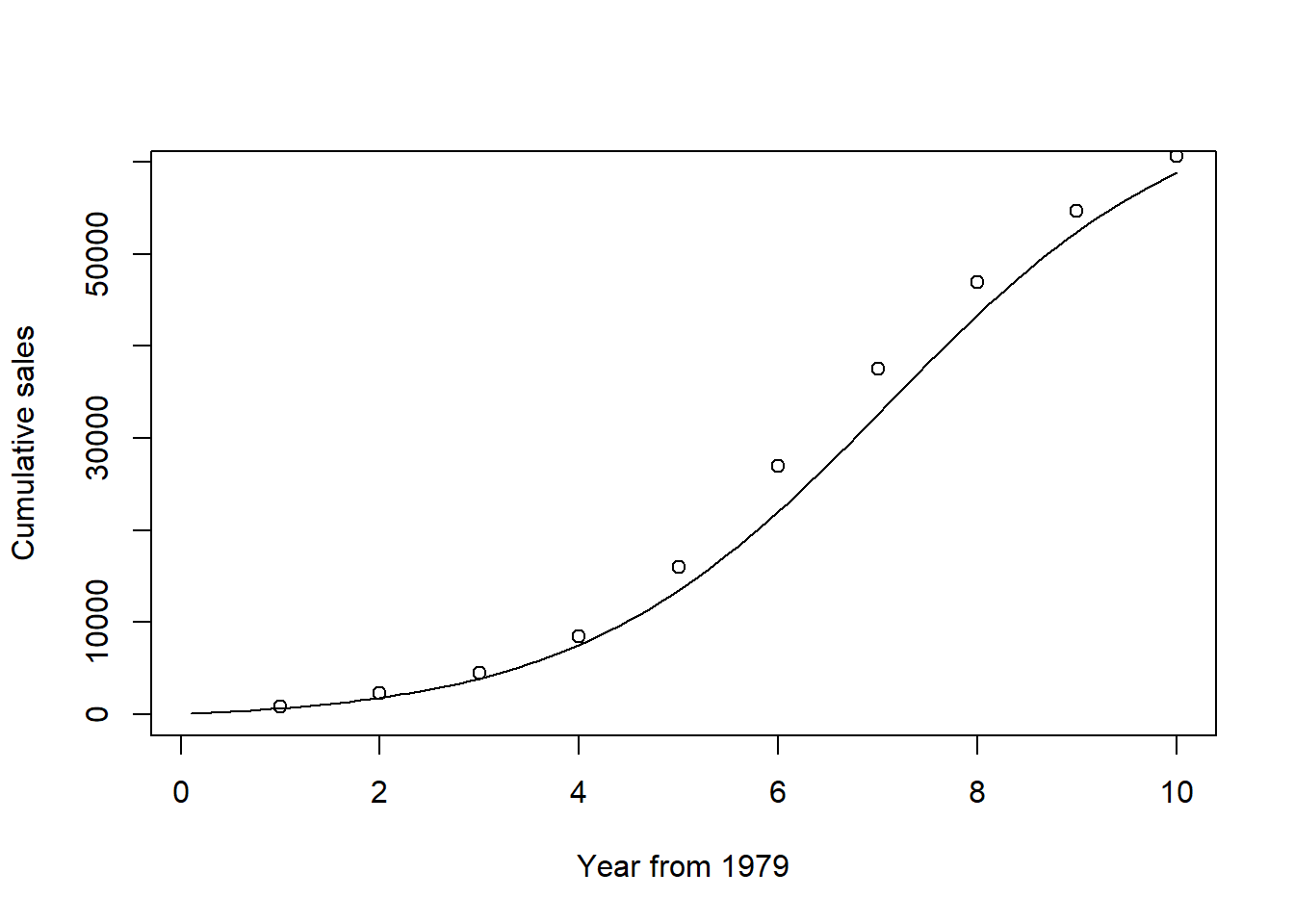Similarly we can forecast using the method given [here] (https://myanalyticsnotes.blogspot.com/2020/03/bass-model-for-forecasting.html) and plot the curve using above method.